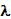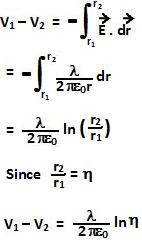## Sunday, May 9, 2010

### Irodov Problem 3.31From Problem 3.22 Eqn 1 we know that the field due to an infinitely long thread with uniform charge densityat a distance r from the thread is given by,directed along the radial direction in the horizontal place perpendicular to the thread (as shown in the figure).

The fact that electric field decays inversely as distance from the thread makes this problem very interesting. This problem cannot be solved using Method 1 as we did in case Problem 3.30. The reason is that every point in space around the thread has an inifinite electric potential energy. In other words it will require an infinite amount of work to bring any charged particle from infinite distance to anywhere close to the thread. Alternatively said, any charged particle left on its own from any point close to thread will acquire an inifinite amount of kinteic energy by the time it reaches far away. This is a direct consequence of the fact that the integral of 1/x diverges to infinity. However, the potential difference between two locations finitely dustance from the thread will be finite.

Consequently, we shall use Method 2 (see 3.30) to solve this problem. The potential difference between two points at distances at distances r1 and r2 is given by,#### 1 comment:

1.hey when u do the integration u get Y/(2(pi)E)*(-ln(r2/r1)=Y/(2(pi)E)*(ln(r1/r2)=Y/(2(pi)E)*(ln(1/n)

I mean check the integration